# OPA2350: Vos and CMRR

Part Number: OPA2350

Hi,

Our customer has made a test of OPA2350 to see the influence of common-mode voltage and the input offset voltage caused by CMRR. And the inverting and non-inverting configuration are shown in TINA, as below. However, there are a few problems. And we really appreciate your help.opa2350 inverting.TSCopa2350 noninverting.TSC

1. In non-inverting configuration, the input signal is 40mV Vpp sinusoidal signal, frequency is 250kHz and 380 kHz. The output signal is shown as below, respectively.

• Why can not the amplifier output get to ±2V?

2. In inverting configuration, the input signal is also 40mV Vpp sinusoidal signal, frequency is 250kHz and 380 kHz. But the output signal is shown as below, respectively.

• Why the output is so much lower than ±2V?
• And this time the output of 250kHz and 380kHz are the same. Is it because that inverting configuration doesn't have common-mode voltage?

3. Is the equation below correct? Or it should be CMRR=ΔVcm/ΔVos ?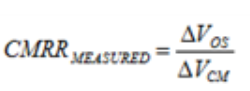4. About CMRR, we have a question. Since CMRR is common-mode rejection, and in non-inverting configuration, the input voltage is common-mode voltage. Does it mean that the input voltage is suppressed in non-inverting configuration?

5. Using the equation below, I can calculate that in non-inverting configuration, ΔVos=20mV*10^(-52/20)=0.05 mV. But the peak value of simulation result is about 14.72 mV. Besides, in inverting configuration, ΔVos is not zero. So we got confusion here.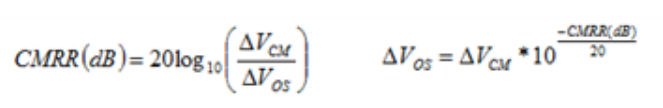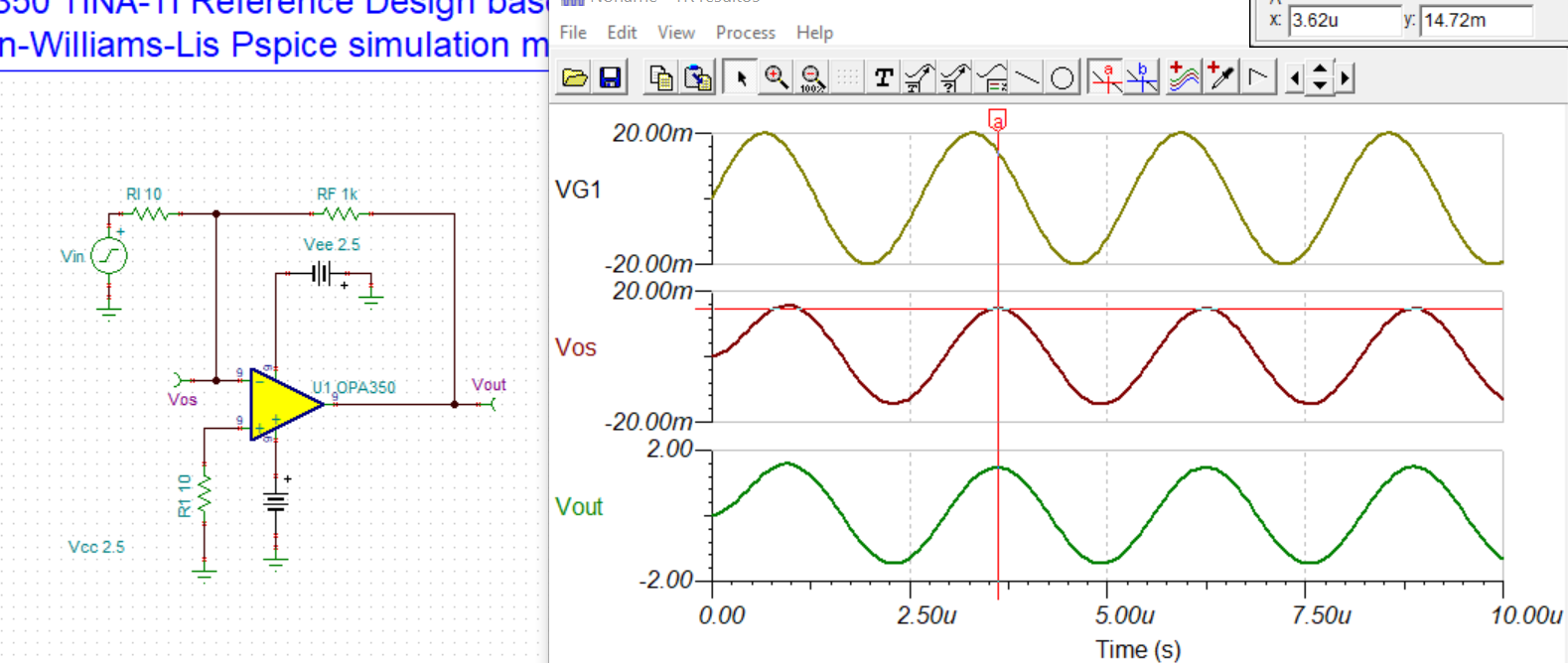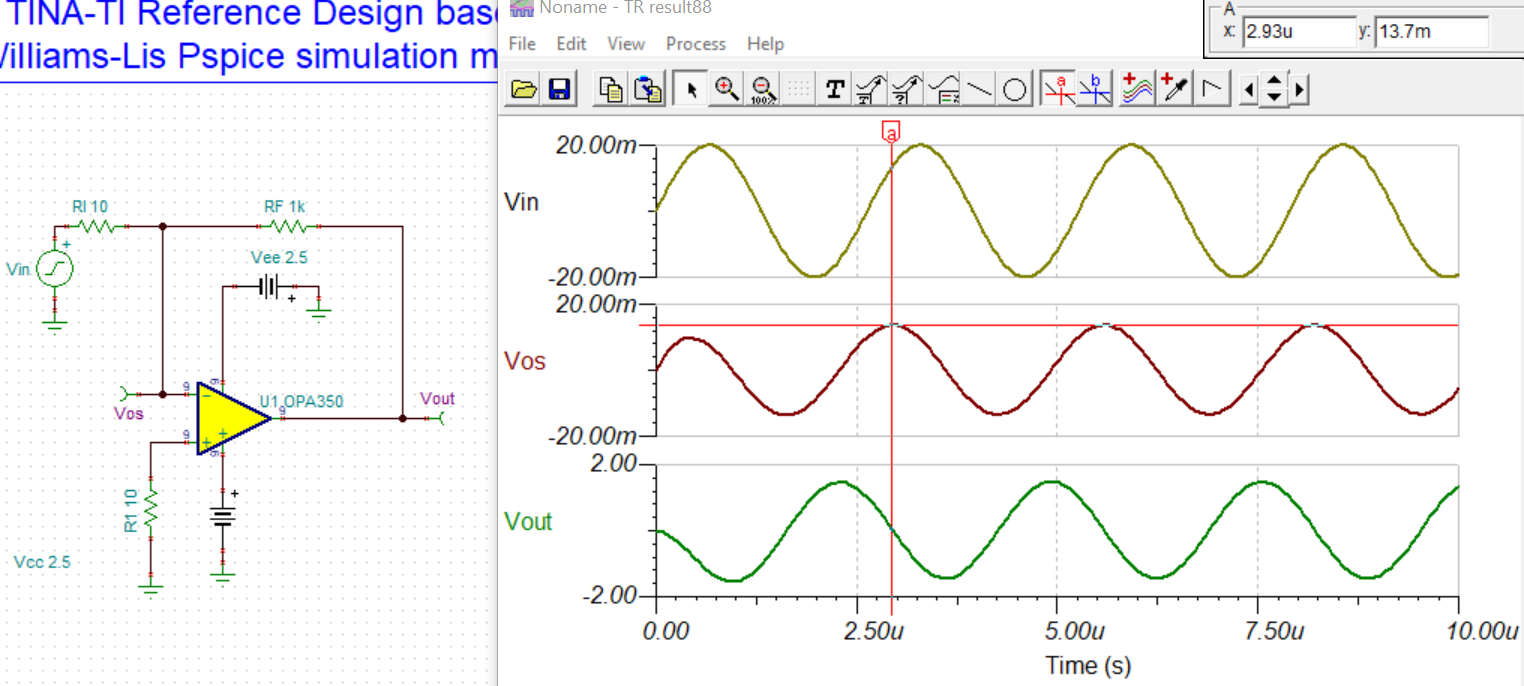Best regards,

Wendy

### 6 Replies

• Hi Wendy,

Q: 1. In non-inverting configuration, the input signal is 40mV Vpp sinusoidal signal, frequency is 250kHz and 380 kHz. The output signal is shown as below, respectively.

• Why can not the amplifier output get to ±2V?
• And why the output of 250kHz and 380kHz are different? Is it because of the ΔVos caused by CMRR

The GBW is specified at 38MHz at Gain=1, At Gain=100, the -3dB point is approx. 380kHz. So it is unable to have gain of 40dB at 380kHz as shown in the image below. at -3dB point, gain = 37dB or Gain=70.8 (10^(37/2) --> output will swing approx 1.42Vpp. 250kB (38.6dB) and 380kB(37.3dB) have different gains, that is why they are different, which the op amp in the roll off region.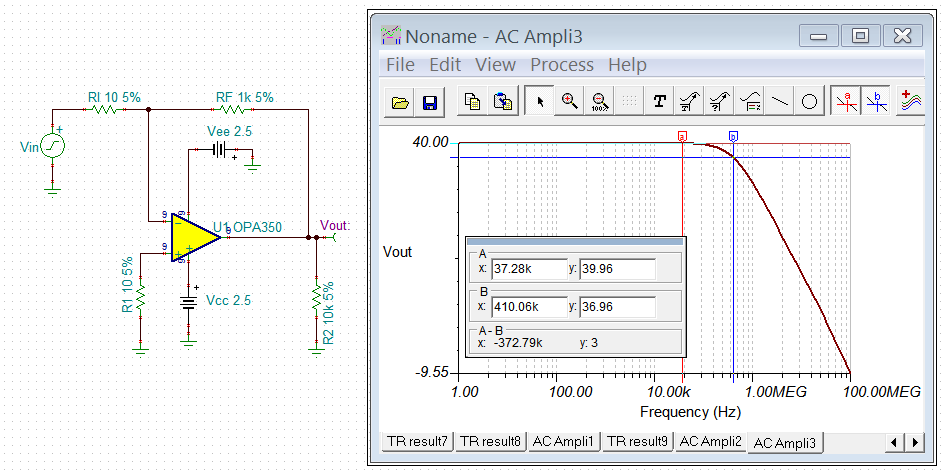Q: 2. In inverting configuration, the input signal is also 40mV Vpp sinusoidal signal, frequency is 250kHz and 380 kHz. But the output signal is shown as below, respectively.

• Why the output is so much lower than ±2V?
• And this time the output of 250kHz and 380kHz are the same. Is it because that inverting configuration doesn't have common-mode voltage?

Same reasons as above, which the op amp starts to attenuate after 50kHz.

Q: 3 & 4.  Is the equation below correct? Or it should be CMRR=ΔVcm/ΔVos ?

The CMRR is defined as the ratio of the powers of the differential gain over the common-mode gain, measured in positive decibels  or Change in Vos/Vcm, which is how TI defines (it is the same thing). Please also see the following videos and application note. Because of log function, sometime you may see they are inverted, but the definition in CMRR is defined as positive.

Regarding to non-inverting CMRR, the V_ and V+ inputs of ideal op amp are the same for ideal op amp.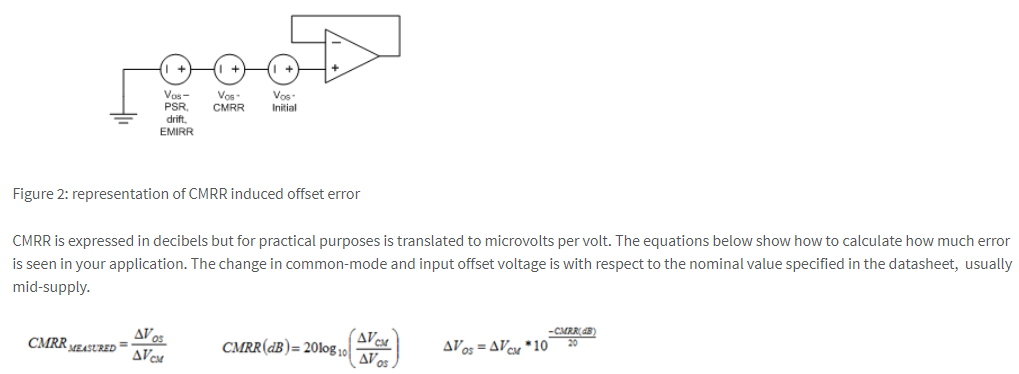https://training.ti.com/ti-precision-labs-op-amps-common-mode-rejection?cu=14685

Q5. Using the equation below, I can calculate that in non-inverting configuration, ΔVos=20mV*10^(-52/20)=0.05 mV. But the peak value of simulation result is about 14.72 mV. Besides, in inverting configuration, ΔVos is not zero. So we got confusion here.

CMRR degrades with frequency.  Please use the method below to calculate the CMRR for a given frequency, also see the reply in Q1 & Q2 due to the gain attenuation at 380kHz.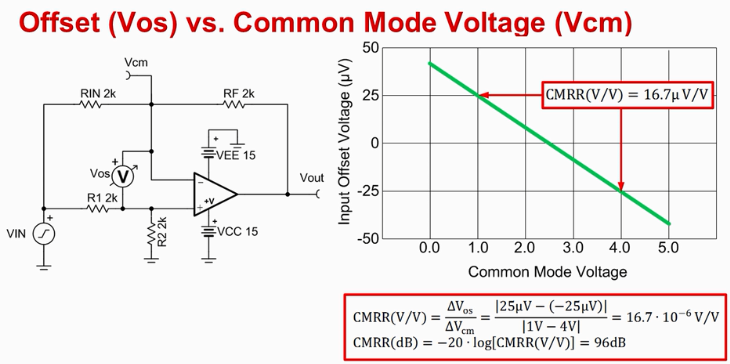Best,

Raymond

• In reply to Raymond Zhang1:

Hi Raymond,

Thank you for your help and support.

1. I can understand that signal at higher frequency has lower gain. But why in the inverting configuration, the output is much lower than that in the non-inverting configuration?

2. How can i configure a circuit using OPA2350 to see the different influence of CMRR on the non-inverting amplifier and the inverting amplifier? (Since in the inverting configuration, the common mode voltage of the op amp is held at the constant DC voltage applied to the non-inverting input of the op amp. Therefore, with a constant in common mode voltage, the inverting topology avoids common mode errors as the input voltage changes.)

And to see that CMRR do degree with frequency? Or you suggest other sockets to test to see the influence?

Best regards,

Wendy

• In reply to Raymond Zhang1:

Hi Raymond,

I use these two circuits to see the difference of ΔVos when input voltage changes, in inverting and non-inverting circuits.inverting.TSCnoninverting.TSC

However, when i change the input voltage from 10mV to 20mV, the ΔVos in non-inverting circuit is lower than that in the inverting circuit.

Best regards,

Wendy

• In reply to Wenxiu Wang:

I put together the AC CMRR and plot it out the simulation, see the attached image. At 380kHz, the CMRR is approx. 51.3dB.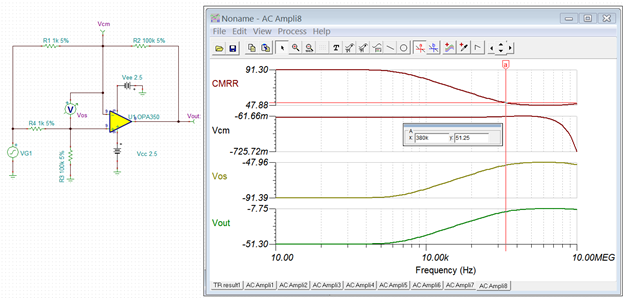/cfs-file/__key/communityserver-discussions-components-files/14/OPA350-CMRR-09152020.TSC

I am still trying to figure out what you are trying to demonstrate. You provided two different circuit. The non-inverting circuit on the left has a gain of 101, and inverting circuit has gain of -100. By the definition shown in the previous attached link, there is only one CMRR calculation. Are we talking about DC CMRR or AC CMRR from your attached file?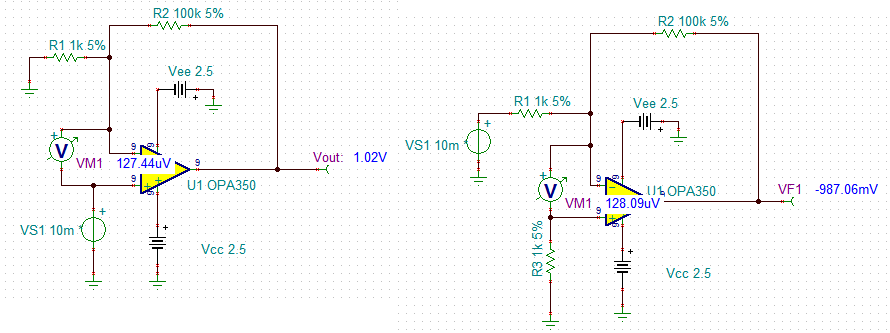There are other CMRR equations, for instance CMRR = 20log|A_cl/A_cm|, where A_cl is gain of a closed loop, and A_cm is the gain of common-mode.  However, if you rearrange the equation, it means the same thing. Please clarify what your customer is trying to obtain, and we will resolve the issue tomorrow.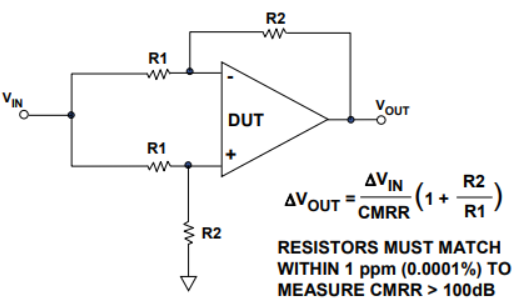Best,

Raymond

• In reply to Raymond Zhang1:

Hi Raymond,

Sorry for the confusion.

1. Since in the inverting configuration, the common mode voltage of the op amp is held at the constant DC voltage applied to the non-inverting input of the op amp. Therefore, with a constant in common mode voltage, the inverting topology avoids common mode errors as the input voltage changes.

Therefore, I am trying to see the different influence of CMRR on the non-inverting amplifier and the inverting amplifier.  DC CMRR and AC CMRR are both OK, as long as it can demonstrate the difference.

2. The circuits I provided, the non-inverting circuit and inverting circuit, is talking about DC CMRR. But when i change the input voltage from 10mV to 20mV, the ΔVos in non-inverting circuit is lower than that in the inverting circuit. I think the result violates this statement ' the inverting topology avoids common mode errors as the input voltage changes.'

3. The other question i asked is that if my circuit is not appropriate to see the different influence of CMRR on the non-inverting amplifier and the inverting amplifier, do you have other suggestions?

• In reply to Wenxiu Wang:

Hi Wendy,

From the previous linked article -> What you need to know about CMRR, I captured the following image, which you also pointed out.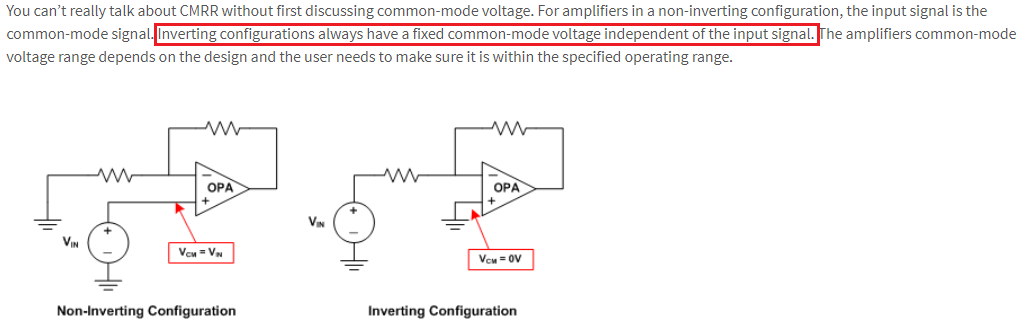Q2: I think the result violates this statement ' the inverting topology avoids common mode errors as the input voltage changes.'

With a very small voltage difference in the voltage offset for the inverting and non-inverting circuits, I think this may be due to the model behavior which may, or may not, be real. They are splitting hairs with a difference of a 1 uV difference!

The key would be to make the bench test measurement of CMRR and see if the real device behaves in the same way as the model. It would be very difficult to measure a 1 uV difference.

Q3. The other question i asked is that if my circuit is not appropriate to see the different influence of CMRR on the non-inverting amplifier and the inverting amplifier, do you have other suggestions?

The difference may actually be due to another parameter changing such as the input bias current. I did a quick check of the input bias current for the two configurations and we can see the bias current is changing between with the two configurations. Enclosed is the simulation so that you can analyze on your own.

/cfs-file/__key/communityserver-discussions-components-files/14/OPA350_5F00_Ib_5F00_vs_5F00_VCM_5F00_01.TSC